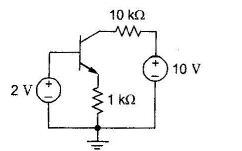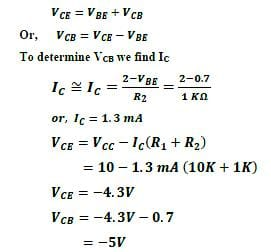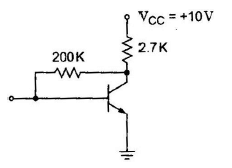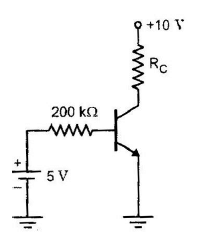# Test: Basic Electronics - 1

## 25 Questions MCQ Test Electrical Engineering SSC JE (Technical) | Test: Basic Electronics - 1

Description
Attempt Test: Basic Electronics - 1 | 25 questions in 50 minutes | Mock test for Electrical Engineering (EE) preparation | Free important questions MCQ to study Electrical Engineering SSC JE (Technical) for Electrical Engineering (EE) Exam | Download free PDF with solutions
QUESTION: 1

### The emitter of a transistor is doped the heaviest because of it :

Solution:

The reason the emitter is the most heavily doped region is because it serves to inject a large amount of charge carriers into the base, which then travels into the collector, so that switching or amplification can occur.

QUESTION: 2

### Voltage power supplies use a high resistance across the output of the supply. That resistance is called

Solution:

Bleeder resistors are standard high value resistors which are used to discharge the capacitor in filter circuit. The discharging of the capacitors is really important because even if the power supply is OFF, a charged capacitor can give a shock to anybody.

QUESTION: 3

### For a NPN bipolar transistor, what is the main stream of current in the base region?​

Solution:

Diffusion current is a current in a semiconductor caused by the diffusion of charge carriers (holes and/or electrons). Diffusion current can be in the same or opposite direction of a drift current.

QUESTION: 4

The current ICBO flows in the

Solution:

ICBO is the collector current with collector junction reverse biased and base open-circuited. ICEO is the collector current with collector junction reverse biased and emitter open-circuited.  ICBO is reverse leakage current going from the Collector to the Base.

QUESTION: 5

Two P-N junction diodes are connected back to back to make a transistor. Which one of the following is correct?

Solution:

This doesn't work because base region is wide and are equally doped, whereas in actual transistor base is very thin and lightly doped. In two discrete diodes connected back-to-back, has 4 doped regions instead of 3. Hence, two diodes connected back-to-back can never be used as a transistor.

QUESTION: 6

The alpha (a) and beta (b) of a transistor are related to each other as

Solution:
QUESTION: 7

ICBO in transistor can be reduced by reducing

Solution:
QUESTION: 8

Ebers-Model of transistor represents two diodes

Solution:
QUESTION: 9

Application of DC voltage to a diode, transistor, or other device to produce a desired mode of operation is called

Solution:
QUESTION: 10

The phenomenon known as "Early Effect" in a bipolar transistor refers to a reduction of the effective base-width caused by

Solution:
QUESTION: 11

Which one of the following specifications is not correct for a common collector amplifier ?

Solution:
QUESTION: 12

In a common collector amplifier the voltage gain is

Solution:

The output voltage on a common-collector amplifier will be in phase with the input voltage, making the common-collector a non-inverting amplifier circuit. The current gain of a common-collector amplifier is equal to β plus 1. The voltage gain is approximately equal to 1 (in practice, just a little bit less).

QUESTION: 13

The transistor configuration which provides highest output impedance is :

Solution:

In Common-Base Transistor, the base-emitter junction JE at input side acts as a forward biased diode. So, the common base amplifier has a low input impedance (low opposition to incoming current). On the other hand, the collector-base junction JC at output side acts somewhat like a reverse biased diode. So the common base amplifier has high output impedance.

Therefore, the common base amplifier provides a low input impedance and high output impedance.

QUESTION: 14

In cutoff region in an N-P-N transistor

Solution:

Whenever we observe the terminals of a BJT and see that the emitter-base junction is not at least 0.6-0.7 volts, the transistor is in the cutoff region. In cutoff, the transistor appears as an open circuit between the collector and emitter terminals. As is seen in 2, this implies Vout is equal to 10 volts.
In cutoff region in an N-P-N transistor VCB is + Ve and VBE is – ve
These are the transistor characteristics.

QUESTION: 15

Consider the following statements in respect of a CC amplifier.
1. It performs a resistance transformation from low to high resistance.
2. Its current gain is close to unity
3. Its voltage gain is close to unity
4. Its frequency range is higher than that of a CE-stage.
Which of the statements is given above are correct?

Solution:
QUESTION: 16

when a transistor is used in switching mode then what is the turn on time ?

Solution:

Turn-on time of the transistor is the time taken by the transistor from the instant the pulse is applied to the instant the transistor switches into the ON state and is the sum of the delay time (td) and rise time (tr).

T(on) tr td
To find out the turn-on time of the transistor, the delay time and rise time have to be calculated.

QUESTION: 17

For transistor, turn-off time is:

Solution:

Turn-off time (TOFF) − The sum of storage time (ts) and fall time (tf) is defined as the Turn-off time.

QUESTION: 18

The voltage divider bias circuit is used in amplifiers quite often becuase it

Solution:
QUESTION: 19

The emitter resistor RE is bypassed by a capacitor in order to

Solution:

Explanation : The main function of the emitter resistor RE bypassed by a capacitor is to improve the stability of the transistor.

QUESTION: 20

For the BJT circuit shown, assume that the b of the transistor is very large and VBE = 0.7 V. The mode of operationSolution:

VBE=0.7V

Input junction (base emitter) junction is forward biased since VBE=0.7

We should find the condition of output junction i.e. C – B junction

Note,Thus, collector base junction is forward biased (since collector is –ve with respect base) Thus, transistor is in saturation

QUESTION: 21

A resistor with colour bands: red-red-red-gold, has the value:

Solution:

Standard EIA Decade Values Table - decade 1 to 10 kΩ The 4 color band resistor color code red-red-red-gold stands for 2.2 kΩ +/-5%, in words: two point two Kiloohms with plus/minus five percent tolerance.

QUESTION: 22

In the circuit as shown b = 99, VBE = 0.6 V, the what are the values of VC and IC corresponding to the operating point?Solution:
QUESTION: 23

A silicon transistor with VBE sat = 0.8 V, b dc = 100 and VCE sat = 0.2 V is used in the circuit shown in given figure What is the minimum value of RC for which transistor is in saturation ?Solution:

Step 1

Apply kvl in 1 loop
5 - IbRb - 0.8 =0
5 - Ib*(200*1000ohm) - 0.8 =0
Base current (Ib)=(5-0.8)/(200*1000ohm)
=(4.2)/(200*1000)
=(2.1)/(100000)

2nd step

we know, collector current (Ic)= beta times of base current
so, Ic=(100*2.1)/(100000)=0.0021

3 step:-

Apply kvl in 2 loop
10 - 0.0021*Rc - 0.2 =0
minimum value of Rc is
Rc=(9.8)/(0.0021)
=4666.66ohm
=4667 ohm

QUESTION: 24

The action of a JFET in its equivalent circuit can best be represented as a

Solution:
QUESTION: 25

Consider the following statements: FETs when compared to BJTs have
1. high input impedance.
2. current flow due to majority carriers.
3. low input impedance
4. current flow due to minority carries.
Which of the statement given above are correct ?

Solution:Use Code STAYHOME200 and get INR 200 additional OFF Use Coupon Code